+关注继续查看

# 要点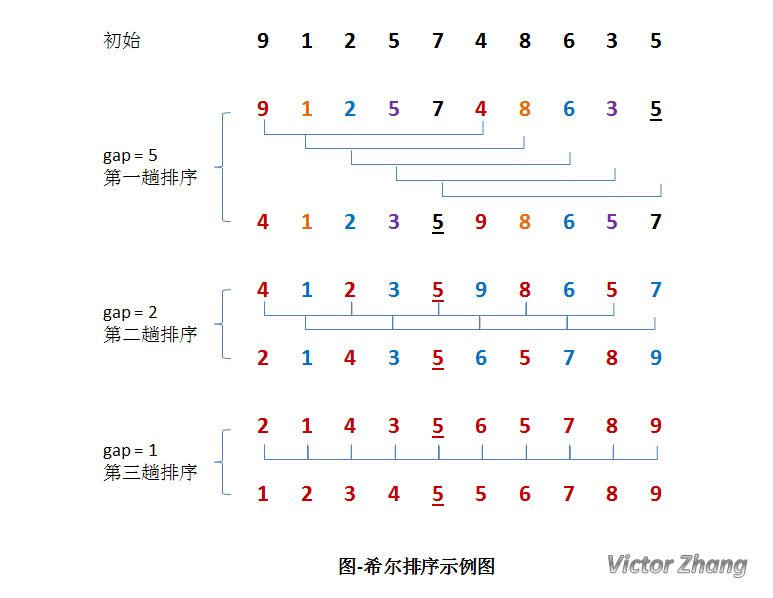public void shellSort(int[] list) {
int gap = list.length / 2;

while (1 <= gap) {
// 把距离为 gap 的元素编为一个组，扫描所有组
for (int i = gap; i < list.length; i++) {
int j = 0;
int temp = list[i];

// 对距离为 gap 的元素组进行排序
for (j = i - gap; j >= 0 && temp < list[j]; j = j - gap) {
list[j + gap] = list[j];
}
list[j + gap] = temp;
}

System.out.format("gap = %d:\t", gap);
printAll(list);
gap = gap / 2; // 减小增量
}
}

# 算法分析

## 希尔排序的算法性能

 排序类别 排序方法 时间复杂度 空间复杂度 稳定性 复杂性 平均情况 最坏情况 最好情况 插入排序 希尔排序 O(Nlog2N) O(N1.5) O(1) 不稳定 较复杂

## 时间复杂度

Donald Shell

 步长序列 最坏情况下复杂度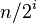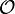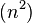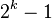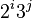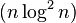# 完整参考代码

## JAVA版本1 package notes.javase.algorithm.sort;
2
3 public class ShellSort {
4     public void shellSort(int[] list) {
5         int gap = list.length / 2;
6
7         while (1 <= gap) {
8             // 把距离为 gap 的元素编为一个组，扫描所有组
9             for (int i = gap; i < list.length; i++) {
10                 int j = 0;
11                 int temp = list[i];
12
13                 // 对距离为 gap 的元素组进行排序
14                 for (j = i - gap; j >= 0 && temp < list[j]; j = j - gap) {
15                     list[j + gap] = list[j];
16                 }
17                 list[j + gap] = temp;
18             }
19
20             System.out.format("gap = %d:\t", gap);
21             printAll(list);
22             gap = gap / 2; // 减小增量
23         }
24     }
25
26     // 打印完整序列
27     public void printAll(int[] list) {
28         for (int value : list) {
29             System.out.print(value + "\t");
30         }
31         System.out.println();
32     }
33
34     public static void main(String[] args) {
35         int[] array = {
36                 9, 1, 2, 5, 7, 4, 8, 6, 3, 5
37         };
38
39         // 调用希尔排序方法
40         ShellSort shell = new ShellSort();
41         System.out.print("排序前:\t\t");
42         shell.printAll(array);
43         shell.shellSort(array);
44         System.out.print("排序后:\t\t");
45         shell.printAll(array);
46     }
47 }
View Code

gap = 5:    4    1    2    3    5    9    8    6    5    7
gap = 2:    2    1    4    3    5    6    5    7    8    9
gap = 1:    1    2    3    4    5    5    6    7    8    9

# 参考资料

《数据结构习题与解析》（B级第3版）

# 相关阅读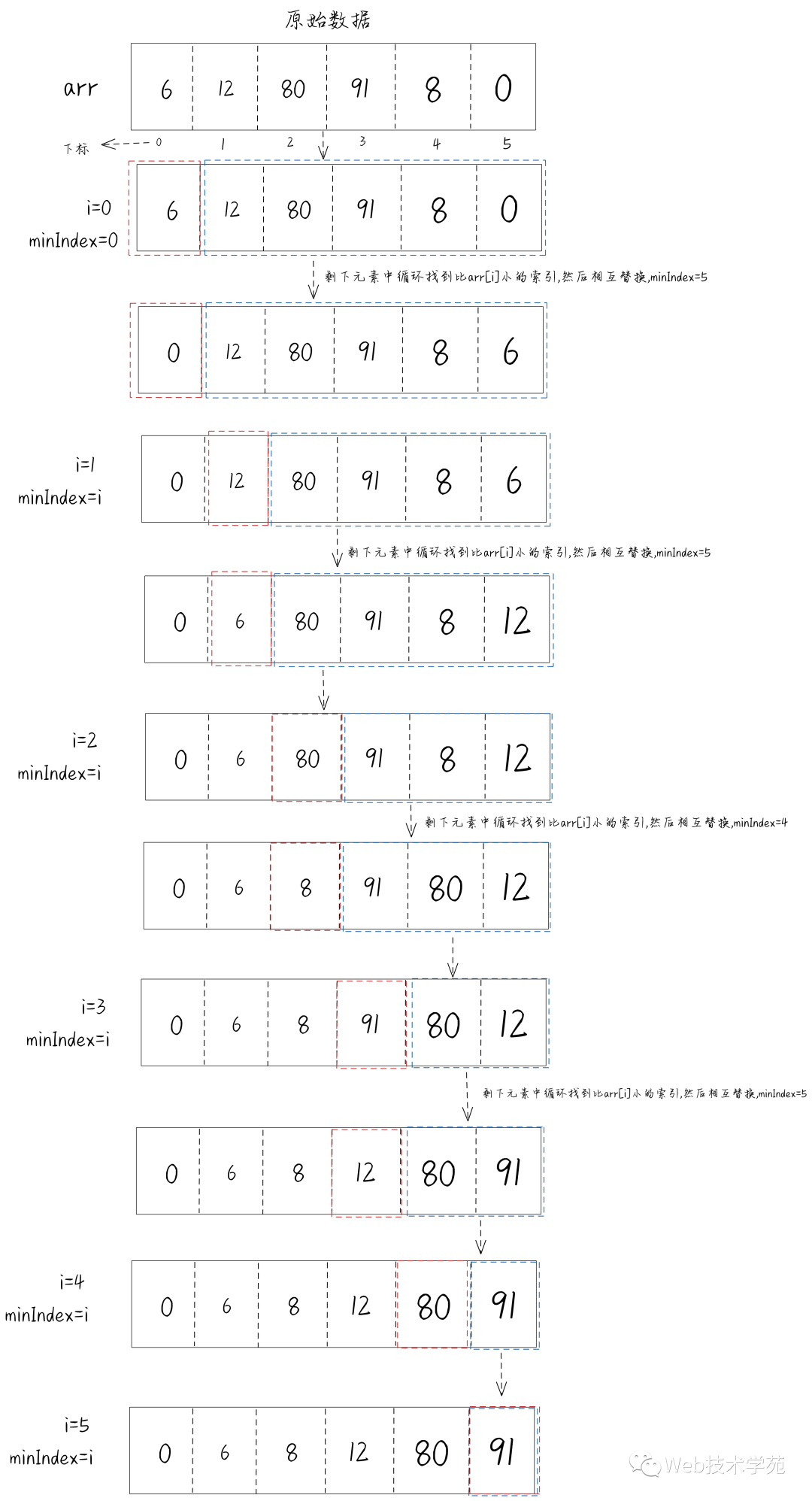39 0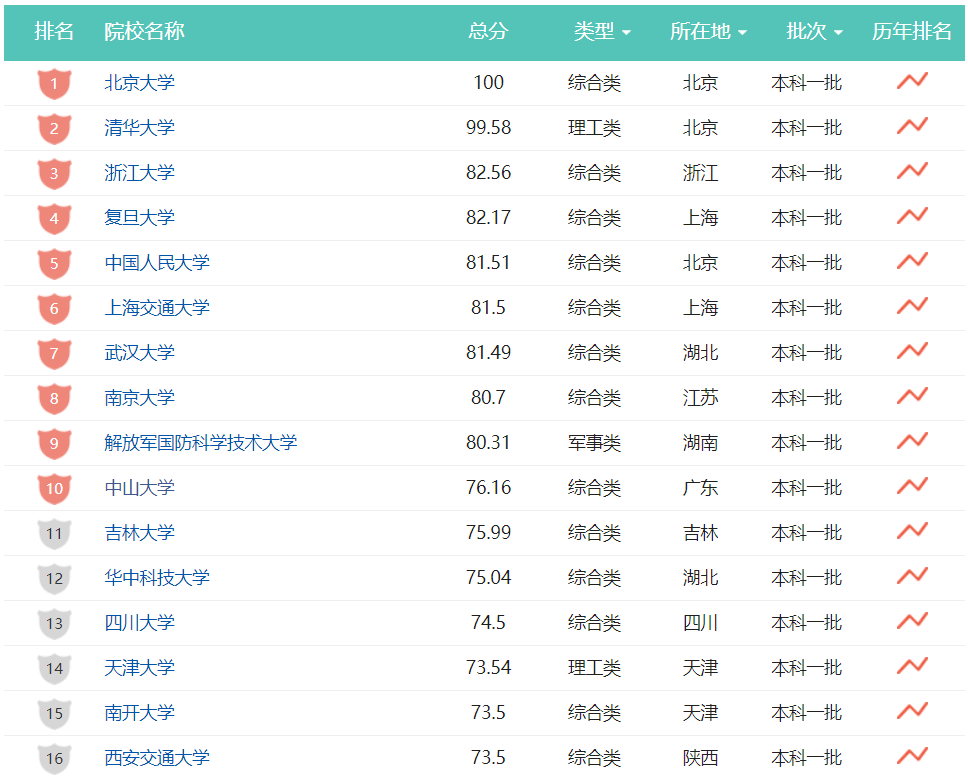42 0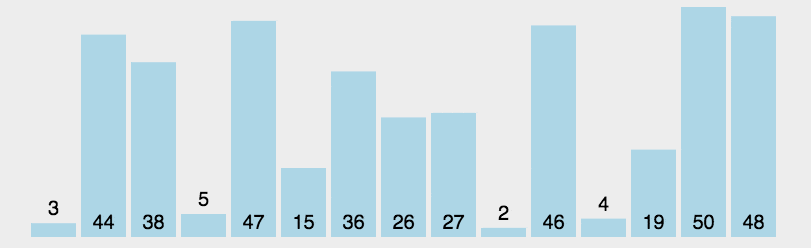40 040 0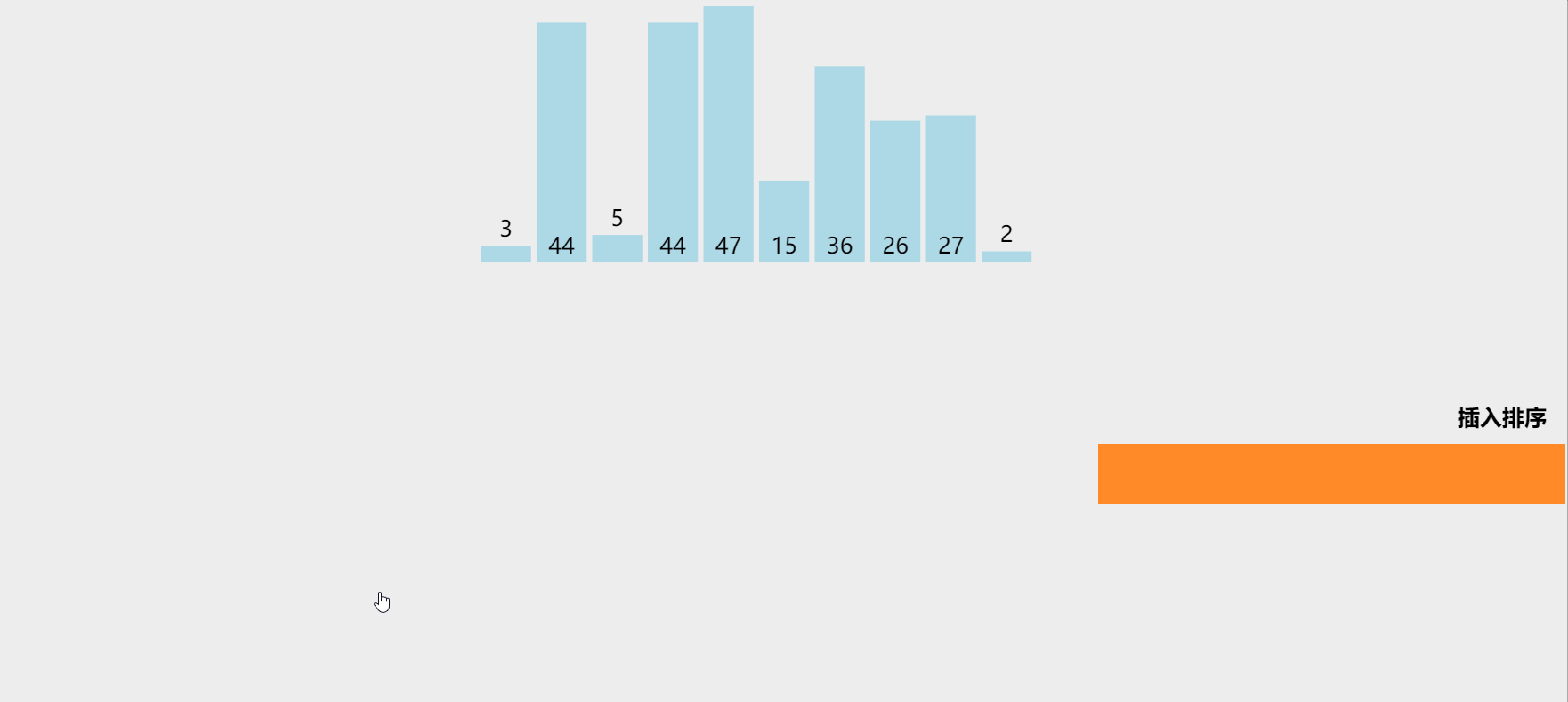40 040 01299 0995 0971 0790 0